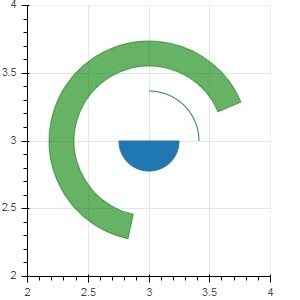# Bokeh - Wedges and Arcs

The arc() method draws a simple line arc based on x and y coordinates, start and end angles and radius. Angles are given in radians whereas radius may be in screen units or data units. The wedge is a filled arc.

The wedge() method has same properties as arc() method. Both methods have provision of optional direction property which may be clock or anticlock that determines the direction of arc/wedge rendering. The annular_wedge() function renders a filled area between to arcs of inner and outer radius.

## Example

Here is an example of arc and wedge glyphs added to Bokeh figure −

```from bokeh.plotting import figure, output_file, show
import math
fig = figure(plot_width = 300, plot_height = 300)
fig.arc(x = 3, y = 3, radius = 50, radius_units = 'screen', start_angle = 0.0, end_angle = math.pi/2)
fig.wedge(x = 3, y = 3, radius = 30, radius_units = 'screen',
start_angle = 0, end_angle = math.pi, direction = 'clock')